1566059103

# Deep Learning Using TensorFlow

In this TensorFlow tutorial for professionals and enthusiasts who are interested in applying Deep Learning Algorithm using TensorFlow to solve various problems.

TensorFlow is an open source deep learning library that is based on the concept of data flow graphs for building models. It allows you to create large-scale neural networks with many layers. Learning the use of this library is also a fundamental part of the AI & Deep Learning course curriculum. Following are the topics that will be discussed in this TensorFlow tutorial:

1. What is TensorFlow
2. TensorFlow Code Basics
3. TensorFlow UseCase

## What are Tensors?

In this TensorFlow tutorial, before talking about TensorFlow, let us first understand what are tensors. **Tensors **are nothing but a de facto for representing the data in deep learning.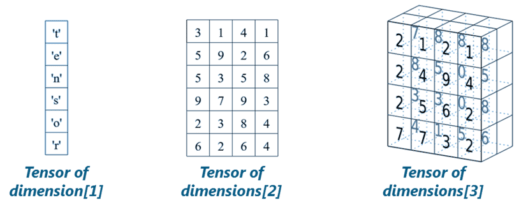As shown in the image above, tensors are just multidimensional arrays, that allows you to represent data having higher dimensions. In general, Deep Learning you deal with high dimensional data sets where dimensions refer to different features present in the data set. In fact, the name “TensorFlow” has been derived from the operations which neural networks perform on tensors. It’s literally a flow of tensors. Since, you have understood what are tensors, let us move ahead in this **TensorFlow **tutorial and understand – what is TensorFlow?

## What is TensorFlow?

**TensorFlow **is a library based on Python that provides different types of functionality for implementing Deep Learning Models. As discussed earlier, the term TensorFlow is made up of two terms – Tensor & Flow: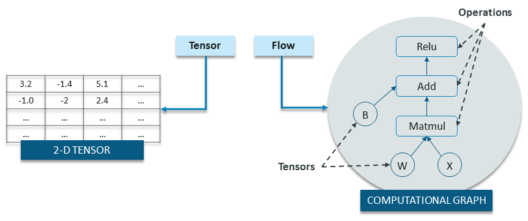In TensorFlow, the term tensor refers to the representation of data as multi-dimensional array whereas the term flow refers to the series of operations that one performs on tensors as shown in the above image.

Now we have covered enough background about TensorFlow.

Next up, in this TensorFlow tutorial we will be discussing about TensorFlow code-basics.

## TensorFlow Tutorial: Code Basics

Basically, the overall process of writing a TensorFlow program involves two steps:

1. Building a Computational Graph
2. Running a Computational Graph

Let me explain you the above two steps one by one:

### 1. Building a Computational Graph

So, what is a computational graph? Well, a computational graph is a series of TensorFlow operations arranged as nodes in the graph. Each nodes take 0 or more tensors as input and produces a tensor as output. Let me give you an example of a simple computational graph which consists of three nodes – a, b & c as shown below: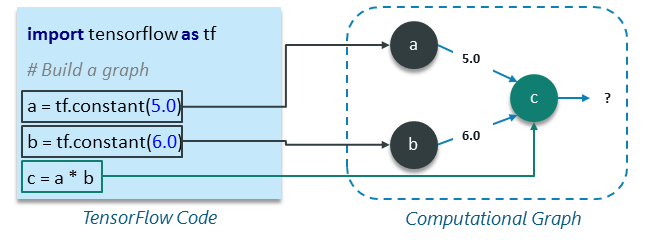#### Explanation of the Above Computational Graph:

**What is TensorFlowTensorFlow Code BasicsTensorFlow UseCase **
Basically, one can think of a computational graph as an alternative way of conceptualizing mathematical calculations that takes place in a TensorFlow program. The operations assigned to different nodes of a Computational Graph can be performed in parallel, thus, providing a better performance in terms of computations.

Here we just describe the computation, it doesn’t compute anything, it does not hold any values, it just defines the operations specified in your code.

### 2. Running a Computational Graph

Let us take the previous example of computational graph and understand how to execute it. Following is the code from previous example:

#### Example 1:

``````import tensorflow as tf

# Build a graph
a = tf.constant(5.0)
b = tf.constant(6.0)
c = a * b

``````

Now, in order to get the output of node c, we need to run the computational graph within a session. Session places the graph operations onto Devices, such as CPUs or GPUs, and provides methods to execute them.

A session encapsulates the control and state of the *TensorFlow *runtime i.e. it stores the information about the order in which all the operations will be performed and passes the result of already computed operation to the next operation in the pipeline. Let me show you how to run the above computational graph within a session (Explanation of each line of code has been added as a comment):

``````# Create the session object
sess = tf.Session()

#Run the graph within a session and store the output to a variable
output_c = sess.run(c)

#Print the output of node c
print(output_c)

#Close the session to free up some resources
sess.close()
Output:
30

``````

So, this was all about session and running a computational graph within it. Now, let us talk about variables and placeholders that we will be using extensively while building deep learning model using TensorFlow.

## Constants, Placeholder and Variables

In TensorFlow, constants, placeholders and variables are used to represent different parameters of a deep learning model. Since, I have already discussed constants earlier, I will start with placeholders.

### Placeholder:

A TensorFlow constant allows you to store a value but, what if, you want your nodes to take inputs on the run? For this kind of functionality, placeholders are used which allows your graph to take external inputs as parameters. Basically, a placeholder is a promise to provide a value later or during runtime. Let me give you an example to make things simpler:

``````import tensorflow as tf

# Creating placeholders
a = tf. placeholder(tf.float32)
b = tf. placeholder(tf.float32)

# Assigning multiplication operation w.r.t. a &amp; b to node mul
mul = a*b

# Create session object
sess = tf.Session()

# Executing mul by passing the values [1, 3] [2, 4] for a and b respectively
output = sess.run(mul, {a: [1,3], b: [2, 4]})
print('Multiplying a b:', output)
Output:
[2. 12.]

``````

#### Points to Remember about placeholders:

**What is TensorFlowTensorFlow Code BasicsTensorFlow UseCase **
Now, let us move ahead and understand – what are variables?

### Variables

In deep learning, placeholders are used to take arbitrary inputs in your model or graph. Apart from taking input, you also need to modify the graph such that it can produce new outputs w.r.t. same inputs. For this you will be using variables. In a nutshell, a variable allows you to add such parameters or node to the graph that are trainable i.e. the value can be modified over the period of a time. Variables are defined by providing their initial value and type as shown below:

``````var = tf.Variable( [0.4], dtype = tf.float32 )

``````

**Note: **
**What is TensorFlowTensorFlow Code BasicsTensorFlow UseCase **
Constants are initialized when you call tf.constant, and their value can never change. On the contrary, variables are not initialized when you call tf.Variable. To initialize all the variables in a TensorFlow program, you must explicitly call a special operation as shown below:

``````init = tf.global_variables_initializer()
sess.run(init)

``````

Always remember that a variable must be initialized before a graph is used for the first time.

Note: TensorFlow variables are in-memory buffers that contain tensors, but unlike normal tensors that are only instantiated when a graph is run and are immediately deleted afterwards, variables survive across multiple executions of a graph.

Now that we have covered enough basics of TensorFlow, let us go ahead and understand how to implement a linear regression model using TensorFlow.

## Linear Regression Model Using TensorFlow

Linear Regression Model is used for predicting the unknown value of a variable (Dependent Variable) from the known value of another variables (Independent Variable) using linear regression equation as shown below: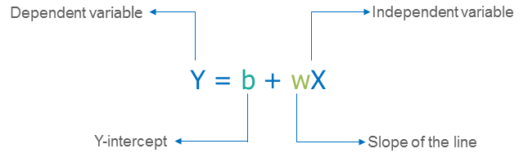Therefore, for creating a linear model, you need:

1. Building a Computational Graph
2. Running a Computational Graph

So, let us begin building linear model using TensorFlow: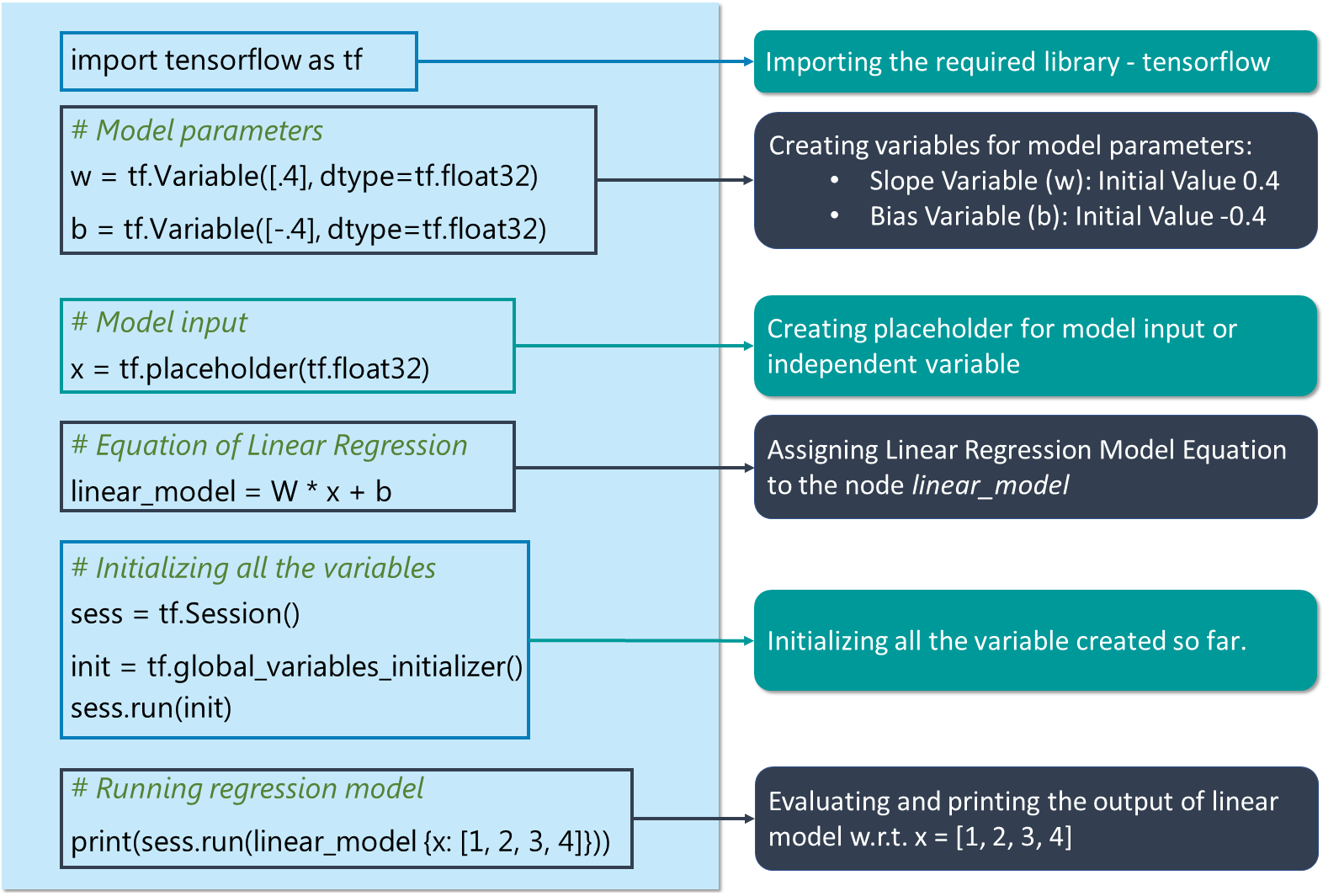Copy the code by clicking the button given below:

``````# Creating variable for parameter slope (W) with initial value as 0.4
W = tf.Variable([.4], tf.float32)

#Creating variable for parameter bias (b) with initial value as -0.4
b = tf.Variable([-0.4], tf.float32)

# Creating placeholders for providing input or independent variable, denoted by x
x = tf.placeholder(tf.float32)

# Equation of Linear Regression
linear_model = W * x + b

# Initializing all the variables
sess = tf.Session()
init = tf.global_variables_initializer()
sess.run(init)

# Running regression model to calculate the output w.r.t. to provided x values
print(sess.run(linear_model {x: [1, 2, 3, 4]}))

``````

Output:

``````[ 0.     0.40000001 0.80000007 1.20000005]

``````

The above stated code just represents the basic idea behind the implementation of regression model i.e. how you follow the equation of regression line so as to get output w.r.t. a set of input values. But, there are two more things left to be added in this model to make it a complete regression model:
**What is TensorFlowTensorFlow Code BasicsTensorFlow UseCase **
Now let us understand how can I incorporate the above stated functionalities into my code for regression model.

### Loss Function – Model Validation

A loss function measures how far apart the current output of the model is from that of the desired or target output. I’ll use a most commonly used loss function for my linear regression model called as Sum of Squared Error or SSE. SSE calculated w.r.t. model output (represent by linear_model) and desired or target output (y) as: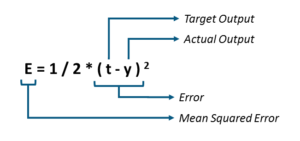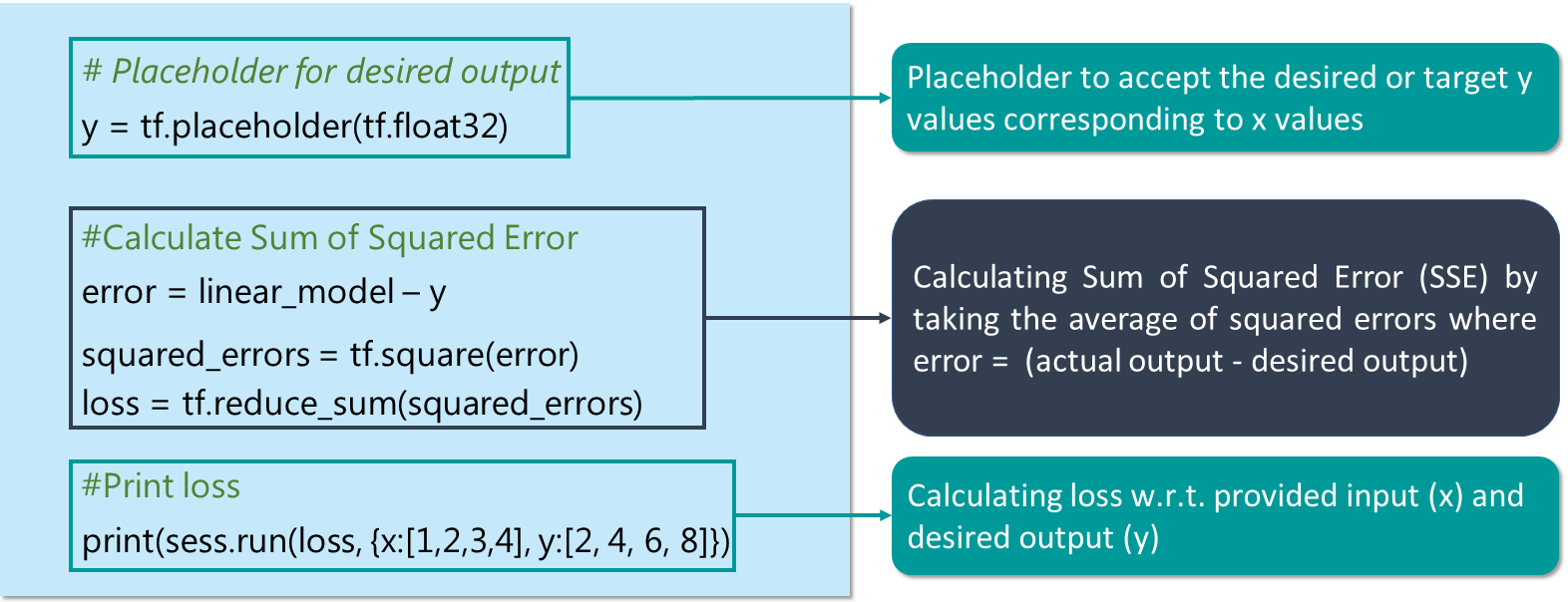``````y = tf.placeholder(tf.float32)
error = linear_model - y
squared_errors = tf.square(error)
loss = tf.reduce_sum(squared_errors)
print(sess.run(loss, {x:[1,2,3,4], y:[2, 4, 6, 8]})

``````
``````Output:
90.24

``````

As you can see, we are getting a high loss value. Therefore, we need to adjust our weights (W) and bias (b) so as to reduce the error that we are receiving.

### tf.train API – Training the Model

TensorFlow provides optimizers that slowly change each variable in order to minimize the loss function or error. The simplest optimizer is gradient descent. It modifies each variable according to the magnitude of the derivative of loss with respect to that variable.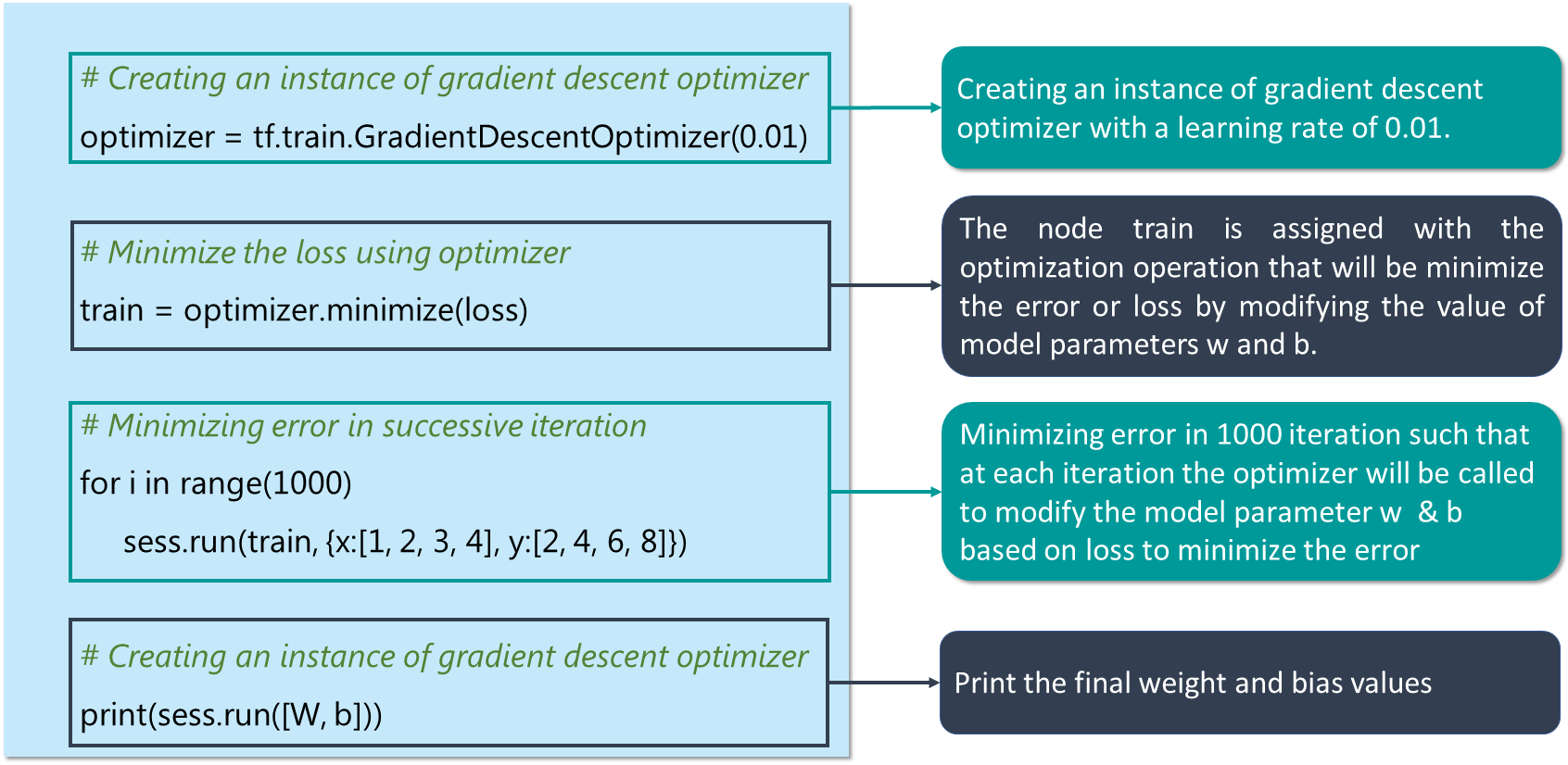``````#Creating an instance of gradient descent optimizer

train = optimizer.minimize(loss)

for i in range(1000):
sess.run(train, {x:[1, 2, 3, 4], y:[2, 4, 6, 8]})
print(sess.run([W, b]))

``````
``````Output:
[array([ 1.99999964], dtype=float32), array([ 9.86305167e-07], dtype=float32)]

``````

So, this is how you create a linear model using TensorFlow and train it to get the desired output.

#tensorflow #python #deep-learning #machine-learning #data-science

## Buddha Community1560826537

``````  File "<ipython-input-18-1e0b90a86e01>", line 19
print(sess.run(linear_model{x: [1, 2, 3, 4]}))
^
SyntaxError: invalid syntax

``````

1560827069

``````  File "<ipython-input-25-f763441d1438>", line 5
print(sess.run(loss, {x:[1,2,3,4], y:[2, 4, 6, 8]})
^
SyntaxError: unexpected EOF while parsing

``````1667425440

## pdf2gerb

Perl script converts PDF files to Gerber format

Pdf2Gerb generates Gerber 274X photoplotting and Excellon drill files from PDFs of a PCB. Up to three PDFs are used: the top copper layer, the bottom copper layer (for 2-sided PCBs), and an optional silk screen layer. The PDFs can be created directly from any PDF drawing software, or a PDF print driver can be used to capture the Print output if the drawing software does not directly support output to PDF.

The general workflow is as follows:

2. Print the top and bottom copper and top silk screen layers to a PDF file.
3. Run Pdf2Gerb on the PDFs to create Gerber and Excellon files.
4. Use a Gerber viewer to double-check the output against the original PCB design.
6. Submit the files to a PCB manufacturer.

Please note that Pdf2Gerb does NOT perform DRC (Design Rule Checks), as these will vary according to individual PCB manufacturer conventions and capabilities. Also note that Pdf2Gerb is not perfect, so the output files must always be checked before submitting them. As of version 1.6, Pdf2Gerb supports most PCB elements, such as round and square pads, round holes, traces, SMD pads, ground planes, no-fill areas, and panelization. However, because it interprets the graphical output of a Print function, there are limitations in what it can recognize (or there may be bugs).

See docs/Pdf2Gerb.pdf for install/setup, config, usage, and other info.

## pdf2gerb_cfg.pm

``````#Pdf2Gerb config settings:
#Put this file in same folder/directory as pdf2gerb.pl itself (global settings),
#or copy to another folder/directory with PDFs if you want PCB-specific settings.
#There is only one user of this file, so we don't need a custom package or namespace.
#NOTE: all constants defined in here will be added to main namespace.
#package pdf2gerb_cfg;

use strict; #trap undef vars (easier debug)
use warnings; #other useful info (easier debug)

##############################################################################################
#configurable settings:
#change values here instead of in main pfg2gerb.pl file

use constant WANT_COLORS => (\$^O !~ m/Win/); #ANSI colors no worky on Windows? this must be set < first DebugPrint() call

#just a little warning; set realistic expectations:
#DebugPrint("\${\(CYAN)}Pdf2Gerb.pl \${\(VERSION)}, \$^O O/S\n\${\(YELLOW)}\${\(BOLD)}\${\(ITALIC)}This is EXPERIMENTAL software.  \nGerber files MAY CONTAIN ERRORS.  Please CHECK them before fabrication!\${\(RESET)}", 0); #if WANT_DEBUG

use constant METRIC => FALSE; #set to TRUE for metric units (only affect final numbers in output files, not internal arithmetic)
use constant APERTURE_LIMIT => 0; #34; #max #apertures to use; generate warnings if too many apertures are used (0 to not check)
use constant DRILL_FMT => '2.4'; #'2.3'; #'2.4' is the default for PCB fab; change to '2.3' for CNC

use constant WANT_DEBUG => 0; #10; #level of debug wanted; higher == more, lower == less, 0 == none
use constant GERBER_DEBUG => 0; #level of debug to include in Gerber file; DON'T USE FOR FABRICATION
use constant WANT_STREAMS => FALSE; #TRUE; #save decompressed streams to files (for debug)
use constant WANT_ALLINPUT => FALSE; #TRUE; #save entire input stream (for debug ONLY)

#DebugPrint(sprintf("\${\(CYAN)}DEBUG: stdout %d, gerber %d, want streams? %d, all input? %d, O/S: \$^O, Perl: \$]\${\(RESET)}\n", WANT_DEBUG, GERBER_DEBUG, WANT_STREAMS, WANT_ALLINPUT), 1);
#DebugPrint(sprintf("max int = %d, min int = %d\n", MAXINT, MININT), 1);

#define standard trace and pad sizes to reduce scaling or PDF rendering errors:
#This avoids weird aperture settings and replaces them with more standardized values.
#(I'm not sure how photoplotters handle strange sizes).
#Fewer choices here gives more accurate mapping in the final Gerber files.
#units are in inches
use constant TOOL_SIZES => #add more as desired
(
#round or square pads (> 0) and drills (< 0):
.010, -.001,  #tiny pads for SMD; dummy drill size (too small for practical use, but needed so StandardTool will use this entry)
.031, -.014,  #used for vias
.041, -.020,  #smallest non-filled plated hole
.051, -.025,
.056, -.029,  #useful for IC pins
.070, -.033,
#    .090, -.043,  #NOTE: 600 dpi is not high enough resolution to reliably distinguish between .043" and .046", so choose 1 of the 2 here
.100, -.046,
.115, -.052,
.130, -.061,
.140, -.067,
.150, -.079,
.175, -.088,
.190, -.093,
.200, -.100,
.220, -.110,
.160, -.125,  #useful for mounting holes
#some additional pad sizes without holes (repeat a previous hole size if you just want the pad size):
.090, -.040,  #want a .090 pad option, but use dummy hole size
.065, -.040, #.065 x .065 rect pad
.035, -.040, #.035 x .065 rect pad
#traces:
.001,  #too thin for real traces; use only for board outlines
.006,  #minimum real trace width; mainly used for text
.008,  #mainly used for mid-sized text, not traces
.010,  #minimum recommended trace width for low-current signals
.012,
.015,  #moderate low-voltage current
.020,  #heavier trace for power, ground (even if a lighter one is adequate)
.025,
.030,  #heavy-current traces; be careful with these ones!
.040,
.050,
.060,
.080,
.100,
.120,
);
#Areas larger than the values below will be filled with parallel lines:
#This cuts down on the number of aperture sizes used.
#Set to 0 to always use an aperture or drill, regardless of size.
use constant { MAX_APERTURE => max((TOOL_SIZES)) + .004, MAX_DRILL => -min((TOOL_SIZES)) + .004 }; #max aperture and drill sizes (plus a little tolerance)
#DebugPrint(sprintf("using %d standard tool sizes: %s, max aper %.3f, max drill %.3f\n", scalar((TOOL_SIZES)), join(", ", (TOOL_SIZES)), MAX_APERTURE, MAX_DRILL), 1);

#NOTE: Compare the PDF to the original CAD file to check the accuracy of the PDF rendering and parsing!
#for example, the CAD software I used generated the following circles for holes:
#CAD hole size:   parsed PDF diameter:      error:
#  .014                .016                +.002
#  .020                .02267              +.00267
#  .025                .026                +.001
#  .029                .03167              +.00267
#  .033                .036                +.003
#  .040                .04267              +.00267
#This was usually ~ .002" - .003" too big compared to the hole as displayed in the CAD software.
#To compensate for PDF rendering errors (either during CAD Print function or PDF parsing logic), adjust the values below as needed.
#units are pixels; for example, a value of 2.4 at 600 dpi = .0004 inch, 2 at 600 dpi = .0033"
use constant
{
HOLE_ADJUST => -0.004 * 600, #-2.6, #holes seemed to be slightly oversized (by .002" - .004"), so shrink them a little
RNDPAD_ADJUST => -0.003 * 600, #-2, #-2.4, #round pads seemed to be slightly oversized, so shrink them a little
SQRPAD_ADJUST => +0.001 * 600, #+.5, #square pads are sometimes too small by .00067, so bump them up a little
TRACE_ADJUST => 0, #(pixels) traces seemed to be okay?
REDUCE_TOLERANCE => .001, #(inches) allow this much variation when reducing circles and rects
};

#Also, my CAD's Print function or the PDF print driver I used was a little off for circles, so define some additional adjustment values here:
#Values are added to X/Y coordinates; units are pixels; for example, a value of 1 at 600 dpi would be ~= .002 inch
use constant
{
CIRCLE_ADJUST_MINY => -0.001 * 600, #-1, #circles were a little too high, so nudge them a little lower
CIRCLE_ADJUST_MAXX => +0.001 * 600, #+1, #circles were a little too far to the left, so nudge them a little to the right
SUBST_CIRCLE_CLIPRECT => FALSE, #generate circle and substitute for clip rects (to compensate for the way some CAD software draws circles)
WANT_CLIPRECT => TRUE, #FALSE, #AI doesn't need clip rect at all? should be on normally?
RECT_COMPLETION => FALSE, #TRUE, #fill in 4th side of rect when 3 sides found
};

use constant SOLDER_MARGIN => +.012; #units are inches

#line join/cap styles:
use constant
{
CAP_NONE => 0, #butt (none); line is exact length
CAP_ROUND => 1, #round cap/join; line overhangs by a semi-circle at either end
CAP_SQUARE => 2, #square cap/join; line overhangs by a half square on either end
CAP_OVERRIDE => FALSE, #cap style overrides drawing logic
};

#number of elements in each shape type:
use constant
{
RECT_SHAPELEN => 6, #x0, y0, x1, y1, count, "rect" (start, end corners)
LINE_SHAPELEN => 6, #x0, y0, x1, y1, count, "line" (line seg)
CURVE_SHAPELEN => 10, #xstart, ystart, x0, y0, x1, y1, xend, yend, count, "curve" (bezier 2 points)
CIRCLE_SHAPELEN => 5, #x, y, 5, count, "circle" (center + radius)
};
#const my %SHAPELEN =
our %SHAPELEN =
(
rect => RECT_SHAPELEN,
line => LINE_SHAPELEN,
curve => CURVE_SHAPELEN,
circle => CIRCLE_SHAPELEN,
);

#panelization:
#This will repeat the entire body the number of times indicated along the X or Y axes (files grow accordingly).
#Display elements that overhang PCB boundary can be squashed or left as-is (typically text or other silk screen markings).
#Set "overhangs" TRUE to allow overhangs, FALSE to truncate them.
use constant PANELIZE => {'x' => 1, 'y' => 1, 'xpad' => 0, 'ypad' => 0, 'overhangs' => TRUE}; #number of times to repeat in X and Y directions

# Set this to 1 if you need TurboCAD support.
#\$turboCAD = FALSE; #is this still needed as an option?

#CIRCAD pad generation uses an appropriate aperture, then moves it (stroke) "a little" - we use this to find pads and distinguish them from PCB holes.
use constant PAD_STROKE => 0.3; #0.0005 * 600; #units are pixels
#convert very short traces to pads or holes:
use constant TRACE_MINLEN => .001; #units are inches
#use constant ALWAYS_XY => TRUE; #FALSE; #force XY even if X or Y doesn't change; NOTE: needs to be TRUE for all pads to show in FlatCAM and ViewPlot
use constant REMOVE_POLARITY => FALSE; #TRUE; #set to remove subtractive (negative) polarity; NOTE: must be FALSE for ground planes

#PDF uses "points", each point = 1/72 inch
#combined with a PDF scale factor of .12, this gives 600 dpi resolution (1/72 * .12 = 600 dpi)
use constant INCHES_PER_POINT => 1/72; #0.0138888889; #multiply point-size by this to get inches

# The precision used when computing a bezier curve. Higher numbers are more precise but slower (and generate larger files).
#\$bezierPrecision = 100;
use constant BEZIER_PRECISION => 36; #100; #use const; reduced for faster rendering (mainly used for silk screen and thermal pads)

# Ground planes and silk screen or larger copper rectangles or circles are filled line-by-line using this resolution.
use constant FILL_WIDTH => .01; #fill at most 0.01 inch at a time

# The max number of characters to read into memory
use constant MAX_BYTES => 10 * M; #bumped up to 10 MB, use const

use constant DUP_DRILL1 => TRUE; #FALSE; #kludge: ViewPlot doesn't load drill files that are too small so duplicate first tool

my \$runtime = time(); #Time::HiRes::gettimeofday(); #measure my execution time

print STDERR "Loaded config settings from '\${\(__FILE__)}'.\n";
1; #last value must be truthful to indicate successful load

#############################################################################################
#junk/experiment:

#use Package::Constants;
#use Exporter qw(import); #https://perldoc.perl.org/Exporter.html

#my \$caller = "pdf2gerb::";

#sub cfg
#{
#    my \$proto = shift;
#    my \$class = ref(\$proto) || \$proto;
#    my \$settings =
#    {
#        \$WANT_DEBUG => 990, #10; #level of debug wanted; higher == more, lower == less, 0 == none
#    };
#    bless(\$settings, \$class);
#    return \$settings;
#}

#use constant HELLO => "hi there2"; #"main::HELLO" => "hi there";
#use constant GOODBYE => 14; #"main::GOODBYE" => 12;

#our @EXPORT_OK = Package::Constants->list(__PACKAGE__); #https://www.perlmonks.org/?node_id=1072691; NOTE: "_OK" skips short/common names

#print STDERR scalar(@EXPORT_OK) . " consts exported:\n";
#foreach(@EXPORT_OK) { print STDERR "\$_\n"; }
#my \$val = main::thing("xyz");
#print STDERR "caller gave me \$val\n";
#foreach my \$arg (@ARGV) { print STDERR "arg \$arg\n"; }``````

Author: swannman
Source Code: https://github.com/swannman/pdf2gerb1618317562

## Top Deep Learning Development Services | Hire Deep Learning Developer

We at Inexture, strategically work on every project we are associated with. We propose a robust set of AI, ML, and DL consulting services. Our virtuoso team of data scientists and developers meticulously work on every project and add a personalized touch to it. Because we keep our clientele aware of everything being done associated with their project so there’s a sense of transparency being maintained. Leverage our services for your next AI project for end-to-end optimum services.

#deep learning development #deep learning framework #deep learning expert #deep learning ai #deep learning services1603735200

## Top 10 Deep Learning Sessions To Look Forward To At DVDC 2020

The Deep Learning DevCon 2020, DLDC 2020, has exciting talks and sessions around the latest developments in the field of deep learning, that will not only be interesting for professionals of this field but also for the enthusiasts who are willing to make a career in the field of deep learning. The two-day conference scheduled for 29th and 30th October will host paper presentations, tech talks, workshops that will uncover some interesting developments as well as the latest research and advancement of this area. Further to this, with deep learning gaining massive traction, this conference will highlight some fascinating use cases across the world.

Here are ten interesting talks and sessions of DLDC 2020 that one should definitely attend:

#### Adversarial Robustness in Deep Learning

By Dipanjan Sarkar

**About: **Adversarial Robustness in Deep Learning is a session presented by Dipanjan Sarkar, a Data Science Lead at Applied Materials, as well as a Google Developer Expert in Machine Learning. In this session, he will focus on the adversarial robustness in the field of deep learning, where he talks about its importance, different types of adversarial attacks, and will showcase some ways to train the neural networks with adversarial realisation. Considering abstract deep learning has brought us tremendous achievements in the fields of computer vision and natural language processing, this talk will be really interesting for people working in this area. With this session, the attendees will have a comprehensive understanding of adversarial perturbations in the field of deep learning and ways to deal with them with common recipes.

Read an interview with Dipanjan Sarkar.

#### Imbalance Handling with Combination of Deep Variational Autoencoder and NEATER

By Divye Singh

**About: **Imbalance Handling with Combination of Deep Variational Autoencoder and NEATER is a paper presentation by Divye Singh, who has a masters in technology degree in Mathematical Modeling and Simulation and has the interest to research in the field of artificial intelligence, learning-based systems, machine learning, etc. In this paper presentation, he will talk about the common problem of class imbalance in medical diagnosis and anomaly detection, and how the problem can be solved with a deep learning framework. The talk focuses on the paper, where he has proposed a synergistic over-sampling method generating informative synthetic minority class data by filtering the noise from the over-sampled examples. Further, he will also showcase the experimental results on several real-life imbalanced datasets to prove the effectiveness of the proposed method for binary classification problems.

#### Default Rate Prediction Models for Self-Employment in Korea using Ridge, Random Forest & Deep Neural Network

By Dongsuk Hong

About: This is a paper presentation given by Dongsuk Hong, who is a PhD in Computer Science, and works in the big data centre of Korea Credit Information Services. This talk will introduce the attendees with machine learning and deep learning models for predicting self-employment default rates using credit information. He will talk about the study, where the DNN model is implemented for two purposes — a sub-model for the selection of credit information variables; and works for cascading to the final model that predicts default rates. Hong’s main research area is data analysis of credit information, where she is particularly interested in evaluating the performance of prediction models based on machine learning and deep learning. This talk will be interesting for the deep learning practitioners who are willing to make a career in this field.

#opinions #attend dldc 2020 #deep learning #deep learning sessions #deep learning talks #dldc 2020 #top deep learning sessions at dldc 2020 #top deep learning talks at dldc 20201593529260

## Few Shot Learning — A Case Study (2)

In the previous blog, we looked into the fact why Few Shot Learning is essential and what are the applications of it. In this article, I will be explaining the Relation Network for Few-Shot Classification (especially for image classification) in the simplest way possible. Moreover, I will be analyzing the Relation Network in terms of:

1. Effectiveness of different architectures such as Residual and Inception Networks
2. Effects of transfer learning via using pre-trained classifier on ImageNet dataset

Moreover, effectiveness will be evaluated on the accuracy, time required for training, and the number of required training parameters.

Please watch the GitHub repository to check out the implementations and keep updated with further experiments.

# Introduction to Few-Shot Classification

In few shot classification, our objective is to design a method which can identify any object images by analyzing few sample images of the same class. Let’s the take one example to understand this. Suppose Bob has a client project to design a 5 class classifier, where 5 classes can be anything and these 5 classes can even change with time. As discussed in previous blog, collecting the huge amount of data is very tedious task. Hence, in such cases, Bob will rely upon few shot classification methods where his client can give few set of example images for each classes and after that his system can perform classification young these examples with or without the need of additional training.

In general, in few shot classification four terminologies (N way, K shot, support set, and query set) are used.

1. N way: It means that there will be total N classes which we will be using for training/testing, like 5 classes in above example.
2. K shot: Here, K means we have only K example images available for each classes during training/testing.
3. Support set: It represents a collection of all available K examples images from each classes. Therefore, in support set we have total N*K images.
4. Query set: This set will have all the images for which we want to predict the respective classes.

At this point, someone new to this concept will have doubt regarding the need of support and query set. So, let’s understand it intuitively. Whenever humans sees any object for the first time, we get the rough idea about that object. Now, in future if we see the same object second time then we will compare it with the image stored in memory from the when we see it for the first time. This applied to all of our surroundings things whether we see, read, or hear. Similarly, to recognise new images from query set, we will provide our model a set of examples i.e., support set to compare.

And this is the basic concept behind Relation Network as well. In next sections, I will be giving the rough idea behind Relation Network and I will be performing different experiments on 102-flower dataset.

The Core idea behind Relation Network is to learn the generalized image representations for each classes using support set such that we can compare lower dimensional representation of query images with each of the class representations. And based on this comparison decide the class of each query images. Relation Network has two modules which allows us to perform above two tasks:

1. Embedding module: This module will extract the required underlying representations from each input images irrespective of the their classes.
2. Relation Module: This module will score the relation of embedding of query image with each class embedding.

Training/Testing procedure:

We can define the whole procedure in just 5 steps.

1. Use the support set and get underlying representations of each images using embedding module.
2. Take the average of between each class images and get the single underlying representation for each class.
3. Then get the embedding for each query images and concatenate them with each class’ embedding.
4. Use the relation module to get the scores. And class with highest score will be the label of respective query image.
5. [Only during training] Use MSE loss functions to train both (embedding + relation) modules.Few things to know during the training is that we will use only images from the set of selective class, and during the testing, we will be using images from unseen classes. For example, from the 102-flower dataset, we will use 50% classes for training, and rest will be used for validation and testing. Moreover, in each episode, we will randomly select 5 classes to create the support and query set and follow the above 5 steps.

That is all need to know about the implementation point of view. Although the whole process is simple and easy to understand, I’ll recommend reading the published research paper, Learning to Compare: Relation Network for Few-Shot Learning, for better understanding.

#deep-learning #few-shot-learning #computer-vision #machine-learning #deep learning #deep learning1595573880

## Deep Reinforcement Learning for Video Games Made Easy

In this post, we will investigate how easily we can train a Deep Q-Network (DQN) agent (Mnih et al., 2015) for Atari 2600 games using the Google reinforcement learning library Dopamine. While many RL libraries exist, this library is specifically designed with four essential features in mind:

• Easy experimentation
• Flexible development
• Compact and reliable
• Reproducible

_We believe these principles makes __Dopamine _one of the best RL learning environment available today. Additionally, we even got the library to work on Windows, which we think is quite a feat!

In my view, the visualization of any trained RL agent is an absolute must in reinforcement learning! Therefore, we will (of course) include this for our own trained agent at the very end!

We will go through all the pieces of code required (which is** minimal compared to other libraries**), but you can also find all scripts needed in the following Github repo.

# 1. Brief Introduction to Reinforcement Learning and Deep Q-Learning

The general premise of deep reinforcement learning is to

“derive efficient representations of the environment from high-dimensional sensory inputs, and use these to generalize past experience to new situations.”

• Mnih et al. (2015)

As stated earlier, we will implement the DQN model by Deepmind, which only uses raw pixels and game score as input. The raw pixels are processed using convolutional neural networks similar to image classification. The primary difference lies in the objective function, which for the DQN agent is called the optimal action-value function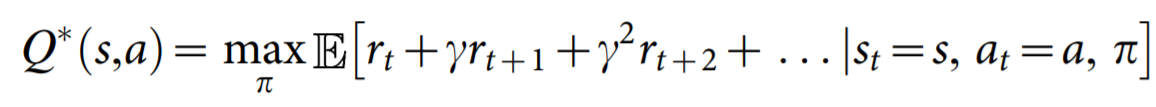where_ rₜ is the maximum sum of rewards at time t discounted by γ, obtained using a behavior policy π = P(a_∣_s)_ for each observation-action pair.

There are relatively many details to Deep Q-Learning, such as Experience Replay (Lin, 1993) and an _iterative update rule. _Thus, we refer the reader to the original paper for an excellent walk-through of the mathematical details.

One key benefit of DQN compared to previous approaches at the time (2015) was the ability to outperform existing methods for Atari 2600 games using the same set of hyperparameters and only pixel values and game score as input, clearly a tremendous achievement.

# 2. Installation

This post does not include instructions for installing Tensorflow, but we do want to stress that you can use both the CPU and GPU versions.

Nevertheless, assuming you are using `Python 3.7.x`, these are the libraries you need to install (which can all be installed via `pip`):

``````tensorflow-gpu=1.15   (or tensorflow==1.15  for CPU version)
cmake
dopamine-rl
atari-py
matplotlib
pygame
seaborn
pandas
``````

#reinforcement-learning #q-learning #games #machine-learning #deep-learning #deep learning